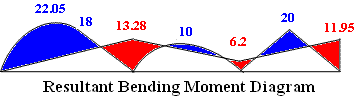Solving indeterminate beam by moment distribution method

Problem 8-1

Use Moment distribution method to find the resultant end moments for the continuous beam shown in figure 8-1(a). Take EI as constant for the beam. Also draw bending moment diagram.Figure 8-1(a)

Solution:

Step 1: The given continuous beam has three spans. Consider each span (AB, BC, and CD) with both ends fixed and calculate the fixed-end moments as follows;

MAB = {wa2 (6L2 - 8aL + 3a2 )}/(12L2 ) = -17.1 kNm

MBA = -{wa3 (4L - 3a}/(12L2 ) = +9.9 kNm

MBC = wL2 /12 = -6.67 kNm

MCB = -wL2 /12 = +6.67 kNm

MCD = PL/8 = -10 kNm

MDC = -PL/8 = +10 kNm

Step 2: Stiffness Coefficients

kAB = 4EI/L = 4EI/5 =0.8EI

kBA = 3EI/L = 3EI/5 =0.6EI

kBC = 4EI/L = 4EI/4 = EI

kCB = 4EI/L = 4EI/4 = EI

kCD = 4EI/L = 4EI/4 = EI

kDC = 4EI/L = 4EI/4 = EI

Step 3: Distribution factors

DAB = 1

DBA = 0.6EI/(0.6EI+EI) = 0.375

DBC = EI/(0.6EI+EI) = 0.625

DCB = EI/(EI+EI) =0.5

DCD = EI/(EI+EI) = 0.5

DDC = 0

Calculation for moment distribution is shown in a systematic way in a tabular form. Each joint is balanced by distributing the unbalanced moment into the connected members  in proportion of distribution factors. These calculation steps for balancing of joints are shown with grey colour. The carry-over moments (half of the balancing moment at near end) are applied on the far end, e.g. carry-over half of the balancing moment from BC to CB.

(In case of mobile device please scroll horizontally to view the full width of table)

 Table for Moment Distribution Joints A B C D Members AB BA BC CB CD DC Distribution Factors 1 0.375 0.625 0.5 0.5 0 Initial Fixed-end Moments -17.1 +9.9 -6.67 +6.67 -10 +10 Balancing +17.1 -3.23x0.375 -1.21 -3.23x0.625-2.02 +3.33x0.5 1.665 +3.33x0.51.665 Carry-over 8.505 0.833 -1.01 0.833 Balancing -9.34x0.375-3.5 -9.34x0.625 -5.84 1.01x0.50.505 1.01x0.5 0.505 Carry-over 0.253 -2.92 0.253 Balancing -0.253x0.375-0.095 -0.253x0.625 -0.158 2.92x0.51.46 2.92x0.5 1.46 Carry-over 0.73 -0.079 0.73 Balancing -0.73x0.375-0.274 -0.73x0.625 -0.456 0.079x0.50.04 0.079x0.5 0.04 Carry-over 0.02 -0.23 0.02 Balancing -0.02x0.375-0.007 -0.02x0.625 -0.012 0.23x0.50.12 0.23x0.5 0.12 Carry-over 0.06 -0.006 0.06 Final End-Moments 0 13.28 -13.27 6.2 -6.2 11.95

Resultant bending moment diagram in figure 8-1(b) is plotted by superposing the span moment diagram and support moment diagram.

Note: Please use bending  moment calculator to get the values of span moments(all values in kNm)

(span moment in blue colour, support moment in red colour)

Figure 8-1(b)

#### Excellent Calculators

Stress Transformation Calculator
Calculate Principal Stress, Maximum shear stress and the their planes

To determine Absolute Max. B.M. due to moving loads.

Bending Moment Calculator
Calculate bending moment & shear force for simply supported beam

Moment of Inertia Calculator
Calculate moment of inertia of plane sections e.g. channel, angle, tee etc.

Reinforced Concrete Calculator
Calculate the strength of Reinforced concrete beam

Moment Distribution Calculator
Solving indeterminate beams

Deflection & Slope Calculator
Calculate deflection and slope of simply supported beam for many load cases

Fixed Beam Calculator
Calculation tool for beanding moment and shear force for Fixed Beam for many load cases

BM & SF Calculator for Cantilever
Calculate SF & BM for Cantilever

Deflection & Slope Calculator for Cantilever
For many load cases of Cantilever

Overhanging beam calculator
For SF & BM of many load cases of overhanging beam

Civil Engineering Quiz
Test your knowledge on different topics of Civil Engineering

Research Papers
Research Papers, Thesis and Dissertation

List of skyscrapers of the world
Containing Tall building worldwide

Forthcoming conferences
Containing List of civil engineering conferences, seminar and workshops

Profile of Civil Engineers
Get to know about distinguished Civil Engineers

Professional Societies
Worldwide Civil Engineers Professional Societies